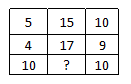# Missing Number Questions for RRB Group-D PDF

0
965

## Missing Number Questions for RRB Group-D PDF

Download Top-15 RRB Group-D Missing Number Questions PDF. RRB GROUP-D Missing Number questions based on asked questions in previous exam papers very important for the Railway Group-D exam.

Question 1: Select the missing number ‘?’ from the given options.

a) 27

b) 35

c) 32

d) 16

Question 2: Select the missing number ‘?’ from the given options.

a) 22

b) 18

c) 14

d) 16

Question 3: Select the missing number ‘?’ from the given options.

a) 26

b) 42

c) 36

d) 34

Question 4: Find the number which should replace ‘?’.

a) 184

b) 110

c) 160

d) 150

Question 5: Select the option which best replaces ‘?’.

a) 113

b) 100

c) 101

d) 106

Question 6: Select the missing number ‘?’ from the given options

a) 10

b) 13

c) 9

d) 14

Question 7: In the following question, select the missing number from the given seriesa) 11

b) 12

c) 15

d) 10

Question 8: In the following question, select the missing number from the given series.

a) 9

b) 8

c) 16

d) 12

Question 9: In the following question select the missing number from the given series

a) 37

b) 47

c) 48

d) 25

Question 10: In the following question, select the missing number from the given series

a) 9

b) 4

c) 8

d) 2

Question 11: In the following question, select the missing number for the given series.

a) Q

b) R

c) S

d) T

Question 12: In the following question select the missing number from the given series

a) 44

b) 48

c) 50

d) 64

Question 13: In the following question, select the missing number from the given series ?

a) 0

b) 4

c) 2

d) 6

Question 14: Find the missing number as per given Series in the table

a) 35

b) 45

c) 25

d) 50

Question 15: In the following question select the missing number of the given series

a) 9

b) 8

c) 6

d) 7

(15 – 6) * 3 = 27
(19 – 8) * 2 = 22
(23 – 15) * 4 = 32
Hence, option C is the right choice.

8*6 / 3 = 16
15 * 2 / 6 = 5
7 * 18 / ? = 9
? = 14
Hence, option C is the right choice.

(3 + 5) * 3 = 24
(9 + 4) * 2 = 26
(5 + 6) * 4 = 44
Hence, option A is the right choice.

In each column, the middle row is twice the sum of the first and the last row.

64 = 2*(19 + 13)

116 = 2*(37 + 21)

Hence, 2*(53+22) = 150

In each column, the third row is the cube of the difference of the first two rows.
$216 = (117 – 111)^3$
$1331 = (80 -69)^3$

Similarly,
$343 = (106 – 99)^3$

$4^2 – 6^2 + 11^2 = 101$
$7^2 – 9^2 + 12^2 = 112$
$?^2 = -8^2 + 5^2 + 139 = 100 = 10^2$
Hence, option A is the right choice.

The sum of three digits in the horizontal row is equal to 30

1) 5 + 15 + 10 = 30

2) 4 + 17 + 9 = 30

3) 10 + x + 10 = 30 ; x = 10

Hence, option D is the correct answer.

The pattern followed is that the number in the last row is obtained by multiplying the other two.

Eg :- $3\times7=21$

$5\times6=30$

Similarly, $8\times1=8$

=> Ans – (B)

The logic followed here is,

$\sqrt{49}$ + $\sqrt{81}$ $\Rightarrow$ 7 + 9 = 16,

$\sqrt{169}$ + $\sqrt{144}$ $\Rightarrow$ 13 + 12 = 25,

$\sqrt{484}$ + $\sqrt{624}$ $\Rightarrow$ 22 + 25 = 47.

Hence, option B is the correct answer.

The pattern followed for $a,b,c$ and $d$ in each column is : $a=(c\times d)-b$

Eg :- $(3\times5)-6=15-6=9$

$(3\times4)-7=12-7=5$

Similarly, $(x\times7)-6=8$

=> $7x=8+6=14$

=> $x=\frac{14}{7}=2$

=> Ans – (D)

The pattern followed is that the alphabets are numbered alphabetically, A=1, B=2, C=3 and so on and then the first alphabet in each column is obtained by :

D=4 and N=14, => $4+14=18\equiv R$

V=22 and B=2, => $22+2=24\equiv X$

Similarly, L=12 and F=6, => $12+6=18\equiv R$

=> Ans – (B)

In each column, the number at the end is the average of other two.

Eg :- $\frac{(36+72)}{2}=\frac{108}{2}=54$

$\frac{(44+88)}{2}=\frac{132}{2}=66$

Similarly, $\frac{(32+64)}{2}=\frac{96}{2}=48$

=> Ans – (B)

The pattern followed is that in each column, the number at the middle is obtained by multiplying the other two.

Eg :- $12\times9=108$

$9\times8=72$

Similarly, $9\times x=36$

=> $x=\frac{36}{9}=4$

=> Ans – (B)

The pattern followed is that in each row, the number at the middle is obtained by multiplying the other two.

Eg :- $6\times7=42$

$8\times4=32$

Similarly, $9\times5=45$

=> Ans – (B)

The sum of each column is same.

Eg :- $6+7+3=16$

and $10+2+4=16$

Similarly, $9+1+x=16$

=> $x=16-10=6$

=> Ans – (C)

We hope this Missing Number Questions  PDF for RRB Group-D Exam will be highly useful for your preparation.